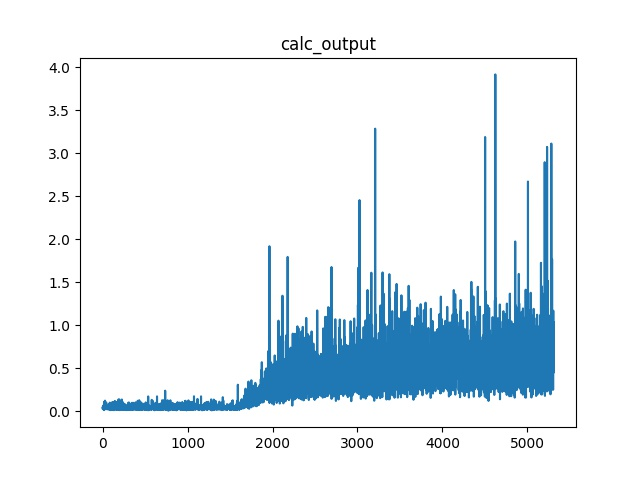# Speed of training slowdown with each epoch

I am training a network with the following model design and training process:

``````class SiameseGCGCN(nn.Module):
def __init__(self, W2V, sent_max_len):
super(SiameseGCGCN, self).__init__()
# network configure
self.embeddings = torch.FloatTensor(W2V.values())
self.num_filter = 100    # number of conv1d filters
self.window_size = 1     # conv1d kernel window size
self.vector_size = 200   # w2v vector size
self.step_size = sent_max_len  # sentence length
nfeat = 2 * self.num_filter
nhid = 50                # graph hidden size
dropout = 0.5

# word2vec input layer
self.embedding = nn.Embedding(self.embeddings.size(0), self.embeddings.size(1))
self.embedding.weight = nn.Parameter(self.embeddings)

# text pair embedding component
self.encoder = nn.Sequential(
nn.Conv2d(in_channels=1,
out_channels=self.num_filter,
kernel_size=(self.window_size, self.vector_size)),
nn.ReLU(),
nn.MaxPool2d(
kernel_size=(self.step_size - self.window_size + 1, 1)))

# graph transformation component
self.gc1 = GraphConvolution(nfeat, nhid)
self.gc2 = GraphConvolution(nhid, nhid)
self.dropout = dropout

# graph aggregation component
self.regressor = nn.Sequential(
nn.Linear(nhid, 1),
nn.Sigmoid())

# index to w2v
x_l = self.embedding(x_l)
x_r = self.embedding(x_r)

# text pair embedding
batch, seq, embed = x_l.size()
x_l = x_l.contiguous().view(batch, 1, seq, embed)
x_r = x_r.contiguous().view(batch, 1, seq, embed)

x_l = x_l.detach()
x_r = x_r.detach()
x_l = self.encoder(x_l)
x_r = self.encoder(x_r)
x_l = x_l.view(-1, self.num_filter)
x_r = x_r.view(-1, self.num_filter)

x_mul = torch.mul(x_l, x_r)
x = torch.cat([x_mul, x_dif], 1)

# graph transformation
x = F.dropout(x, self.dropout, training=self.training)

# graph aggregation
x = torch.mean(x, dim=0)
out = self.regressor(x)

return out
``````

and the following training method:

``````def train(epoch):
t = time.time()
outputs = []
loss_train = 0.0
for i in idx_train:
# gc.collect()  # !!!!!!!!!!!!!!!!!!
print(i)
feature_l = Variable(features_l[i])
feature_r = Variable(features_r[i])
# g_feature = Variable(g_features[i])
label = Variable(torch.FloatTensor([labels[i]]))  # must add []
model.train()
output = model(feature_l, feature_r, adj)  # what if batch > 1 ?
outputs.append(output.data)
loss = nn.BCELoss()(output, label)
loss_train += loss.data  #  !!!!! notice, must use .data, otherwise memory keep increasing. The graph keep increasing.
loss.backward()
optimizer.step()
loss_train = loss_train / len(idx_train)
acc_train = bc_accuracy(torch.stack(outputs),
labels[torch.LongTensor(idx_train)])

# Evaluate validation set performance separately,
# deactivates dropout during validation run.
loss_val, acc_val = test(model, features_l, features_r, g_features, adjs, labels, idx_val)

print('Epoch: {:04d}'.format(epoch + 1),
'loss_train: {:.4f}'.format(loss_train),
'acc_train: {:.4f}'.format(acc_train),
'loss_val: {:.4f}'.format(loss_val),
'acc_val: {:.4f}'.format(acc_val),
'time: {:.4f}s'.format(time.time() - t))
``````

However, I found the training time increases with each for iteration.
At the beginning, each second can process about 10 samples. But after about 1500~2000 iterations, the time increased to about 1 second per sample, and will keep increasing.

I analyzed the time for each step in the for loop of the train method. I found that the following two steps’ time are increasing:

``````        output = model(feature_l, feature_r, adj)  # what if batch > 1 ?
optimizer.step()
``````

``````        optimizer = optim.Adam(parameters,
lr=args.lr, weight_decay=args.weight_decay)
``````

I debugged for a few days and try to find similar problems on this forum and google sites, but haven’t got the solution for my case. So I want to ask why this happen, what caused my network training speed slows down? Thanks!

This image shows the speed of optimizer.step():This image shows the speed of calculate output:This image shows the speed of backward step:For other steps, seems the speed don’t change too much with different loop iterations.

1 Like

Hi @bang_liu, did you find the source of your slow down? I may have a similar problem…

Hi @Thomas_Wolf, I haven’t figured out at that time, I revised my code and didn’t remember what happened after that…

Did anyone find what was going on here. I am having maybe the same problem. Early epochs of training take ~27 s, while after 40 epochs times can be up to ~150 s.

The speed (and change thereof) for me is also quite dependent upon the learning rate. Also I have mostly been using the optimiser Adam, but I also get it with SGD.

I have looked quite a bit into what effects this, and roughly where the slow is coming in, so could post more if there are people who are curious.